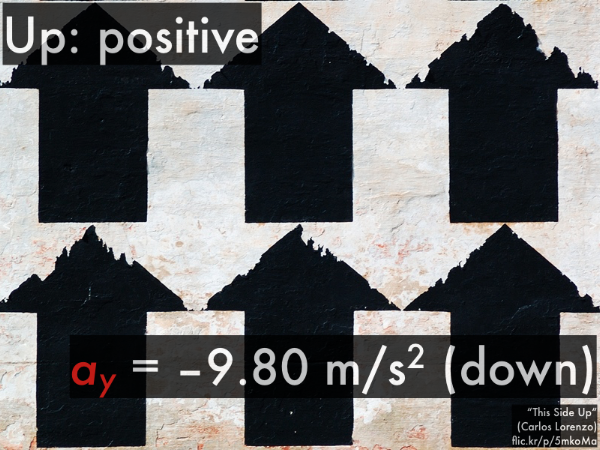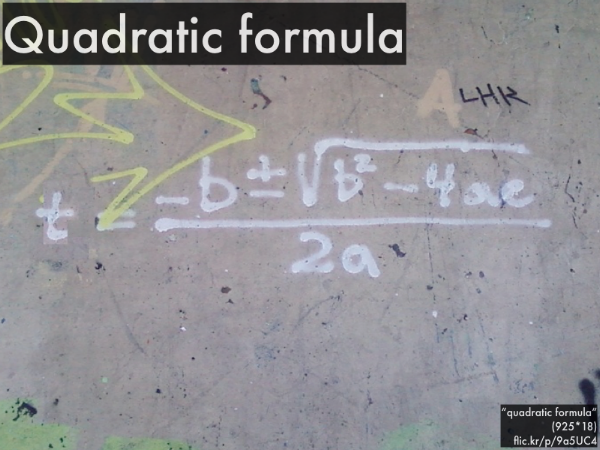20150902

Physics presentation: free fallThis is only just a little disturbing, but I can't stop watching this. (Movie link: "experiment with apples.")So let's try to analyze this and similar types of motion--free fall--using graphs and equations from our one-dimensional motion toolbox.Our working definition of free fall is an object that is subject only to the force of gravity. Nothing in contact with it, nor any drag (although we often make the assumption that drag forces are negligible).So let's take a look at how the kinematic equations turn out for free fall motion.We'll consider the convention where up is the positive vertical direction. (If you're the type that likes to call down positive, you're just being contrary and weird.) Since an object in free fall is only experiencing the force of gravity, then it will experience the acceleration due to gravity (of magnitude 9.80 m/s2), which is directed downwards, and thus requires an obligatory negative sign.Note that our vertical motion equations will then have a vertical acceleration ay = –9.80 m/s2. Again, that obligatory negative sign (also we'll assume that the starting position is y0 = 0 m at t0 = 0 s).Also the quadratic formula will often be useful as well for free fall.Let's take a look at every conceivable vertical velocity vy vs. t graph there could possibly be for any type of free fall situation.But don't worry, there are only three possible graphs. Notice that they have all have the same negative slope--and since the slope of a velocity versus time graph is acceleration, these graphs all have the same acceleration ay = –9.80 m/s2. The only difference between these graphs is the initial vertical velocity v0y, whether positive (thrown upwards), zero (and thus released from rest), or negative (and thus thrown downwards).

So let's take a look at some situations, and decide which free fall graph best describes them (assuming we can neglect drag).A boy steps off of a ledge (with no initial vertical velocity) and splashes into the water below.
The vy(t) graph has __________ initial velocity v0y.
(A) a negative.
(B) zero.
(C) a positive.
(D) (Unsure/guessing/lost/help!)

The vertical distance traveled is __________ the magnitude of the vertical displacement.
(A) less than.
(B) equal to.
(C) greater than.
(D) (Unsure/guessing/lost/help!)A ball is thrown and released downwards from the top of a building, and hits the ground below.
The vy(t) graph has __________ initial velocity v0y.
(A) a negative.
(B) zero.
(C) a positive.
(D) (Unsure/guessing/lost/help!)

The vertical distance traveled is __________ the magnitude of the vertical displacement.
(A) less than.
(B) equal to.
(C) greater than.
(D) (Unsure/guessing/lost/help!)A hat is thrown and released upwards into the air and lands on the grass below.
The vy(t) graph has __________ initial velocity v0y.
(A) a negative.
(B) zero.
(C) a positive.
(D) (Unsure/guessing/lost/help!)

The vertical distance traveled is __________ the magnitude of the vertical displacement.
(A) less than.
(B) equal to.
(C) greater than.
(D) (Unsure/guessing/lost/help!)